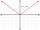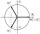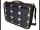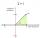Log

Calculate value of expression log |3 +7i +5i2| .

Result

x =  0.86

Solution:Leave us a comment of this math problem and its solution (i.e. if it is still somewhat unclear...):Be the first to comment!To solve this verbal math problem are needed these knowledge from mathematics:

Try our complex numbers calculator.

Next similar examples:

1. Null pointsCalculate the roots of the equation: ?
2. ReciprocalCalculate reciprocal of z=0.8-1.8i:
3. De Moivre's formulaThere are two distinct complex numbers z such that z3 is equal to 1 and z is not equal 1. Calculate the sum of these two numbers.
4. Linear imaginary equationGiven that ? "this is z star" Find the value of the complex number z.
5. GainFind the gain whenever the output power is 21x the input power.
6. Intercept with axisF(x)=log(x+4)-2, what is the x intercept
7. LogarithmDetermine the number whose decimal logarithm is -3.8.
8. Logif ?, what is b?
9. CalculationHow much is sum of square root of six and the square root of 225?
10. Imaginary numbersFind two imaginary numbers whose sum is a real number. How are the two imaginary numbers related? What is its sum?
11. Im>0?Is -10i a positive number?
12. SequenceBetween numbers 1 and 53 insert n members of the arithmetic sequence that its sum is 702.
13. Theorem proveWe want to prove the sentence: If the natural number n is divisible by six, then n is divisible by three. From what assumption we started?
14. Complex number coordinatesWhich coordinates show the location of -2+3i
15. Is complexAre these numbers 2i, 4i, 2i + 1, 8i, 2i + 3, 4 + 7i, 8i, 8i + 4, 5i, 6i, 3i complex?
16. Six termsFind the first six terms of the sequence a1 = -3, an = 2 * an-1
17. 75th percentile (quartille Q3)Find 75th percentile for 30,42,42,46,46,46,50,50,54